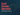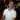# Excel Formula Operators

April 11, 2018

We all use Excel formulas, but have you ever wondered what all of the special characters mean in the syntax? From math operations, to Excel Table formulas, there are plenty of special characters to consider. In this post, we discuss all of the different ways Excel formulas use special characters, how they work, and how to use them.

By the way, if you aren’t familiar with formulas, definitely check this out first.

All of the special characters are laid out below. You can copy and paste the examples into Excel to check them out.

Let’s jump right in.

## What is an Operator?

No, no, not that kind of operator (by the way, if you haven’t seen “The IT Crowd” please stop whatever you’re doing and go watch it - it’s hilarious).

All of these “special characters” I referenced in the intro are actually called operators. They are called operators because they operate on the operands beside them.

As a simple example, here is a simple math function:

``=1+2``

Here, the `+` is the operator (in this case, the addition operator) and the `1` and `2` are the operands. Some more info about this can be found here.

The following sections introduce the different types of operations and the operators that belong to them.## Math Operations

Excel comes with basic mathematical operators. This is pretty straightforward, but it’s important to cover.

Character Meaning Example
`+` Addition `=1+2`
`-` Subtraction `=2-1`
`*` Multiplication `=2*2`
`/` Division `=4/2`
`^` Power `=2^2`
`%` Percent `30%`

## Logic Operations

Excel also comes with logical operators. This is useful when making comparisons inside an `IF` statement.

Character Meaning Example
`<` Less than `=IF(1<2,"Yes","No")`
`<=` Less than or equal to `=IF(2<=2,"Yes","No")`
`>` Greater than `=IF(2>1,"Yes","No")`
`>=` Greater than or equal to `=IF(2>=2,"Yes","No")`
`<>` Not equal to `=IF(1<>2,"Yes","No")`
`=` Equal to `=IF(1=1,"Yes,"No")`

## Grouping Operators

I wasn’t sure what to call this section, but it discusses the use of parenthesis in Excel formulas.

Character Meaning Example
`( )` Used to group operations together to override standard operator precedence. `=(1+1)*5` vs `=1+1*5`

## String Operations

While there are plenty of string functions, there are just a few string operators.

Character Meaning Example
`"` Start or end a string `="This is a string"`
`&` Concatenate (join) two strings. Numbers can be converted to strings. `="This is "&1&" string."`
`'` Treat number as text. Though not a formula, this is important to remember. `'00123`
`'` Also treat a formula as text. `'=A1+A2`

## Cell / Range Operators

What good would Excel be without the awesome ability to work with cells and ranges? Here are all of the relative characters when working with cells and ranges.

Character Meaning Example
`A-XFD` Column specifier. As in `A1` or `XFD1`. `=A1`
`1-1048576` Row specifier. As in `A1` or `A2`. `=A1`
`:` Range specifier (see below for more info). Usually represented as top-left to bottom right. `=SUM(A1:B5)`
`\$` Reference lock. Sets a range to be an absolute reference. See here for more info. `=SUM(\$A1:\$B5)`
space A space intersects two ranges to produce a common range between them. `=SUM(A1:B2 B2:C3)`

### Range Specifier Rules (mini post)

Let’s touch on the Range Specifier a little further. When specifying a range, you can include columns and/or rows.

For example, specify an entire column:

``=SUM(A:A)``

Or specify a range of columns:

``=SUM(A:D)``

You can also specify an entire row:

``=SUM(1:1)``

Or a range of rows:

``=SUM(1:10)``

However, you can’t mix and match these rules. For example, you can’t do the following:

``=SUM(A:1)``

This might read “sum all values in column `A` and row `1`.” Or this might be a typo and meant to be just `A1`.

However, in some functions, you can specify multiple ranges. So, to sum column `A` and row `1` values, you can do the following:

``=SUM(A:A,1:1)``

This is because some functions accept multiple range arguments, and the `SUM` function is one of them:

You can tell this accepts multiple formulas because of the ellipses at the end:

``=SUM(number1, [number2], ...)``

To summarize this point, if you need to specify multiple ranges, separate each range with a comma and be sure that the function your using allows multiple ranges.

## Array Operations

There are two ways to think about array operations in Excel: array formulas and array constants. The table below describes the different characters that work with arrays.

Character Meaning Example
`{ }` When surrounding a formula, this represents an array formula (entered with Ctrl+Shift+Enter).  `{=AVERAGE(IF(A1:A5>20,A1:A5))}`
`{ }` When not surrounding a formula, this represents an array constant. `={1,2;3,4}`
`,` Separates values horizontally in an array constant. `={1,2}`
`;` Separates values vertically in an array constant. `={1;2}`

## Excel Table Operations

Excel Table operations are basically known as Structured References. I did an entire post on structured references with a nice infographic here.

Character Meaning Example
`[ ]` Grants access to special items within the table (like #Headers). `=[#Headers]`
`#` Specifies a special item within the table. `=[#Data]`
`@` Specifies the current row of the formula. `=[@Cost]*[@Markup]`

## Wrap Up

If you made it to this point, congrats! There is a lot to know when it comes to Excel formulas and I’m sure that I’ll find myself coming back to this page for my own reference, and I hope you enjoyed this enough that you’ll use this as a reference for yourself, too.

By the way, if I’m missing any special characters or just want to ask a question about these characters please comment below!

Wow, you read the whole article! You know, people who make it this far are true learners. And clearly, you value learning. Would you like to learn more about Excel? Please consider supporting me by buying me a coffee (it takes a lot of coffee to write these articles!).Written by Joseph who loves teaching about Excel.